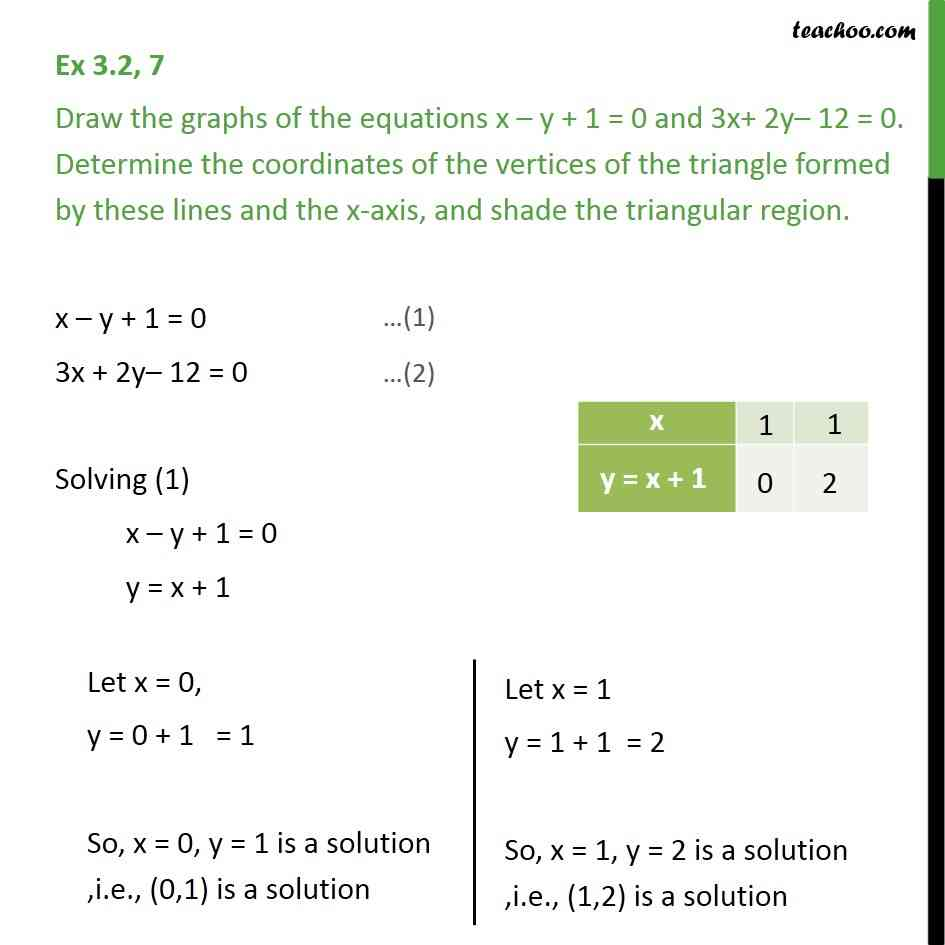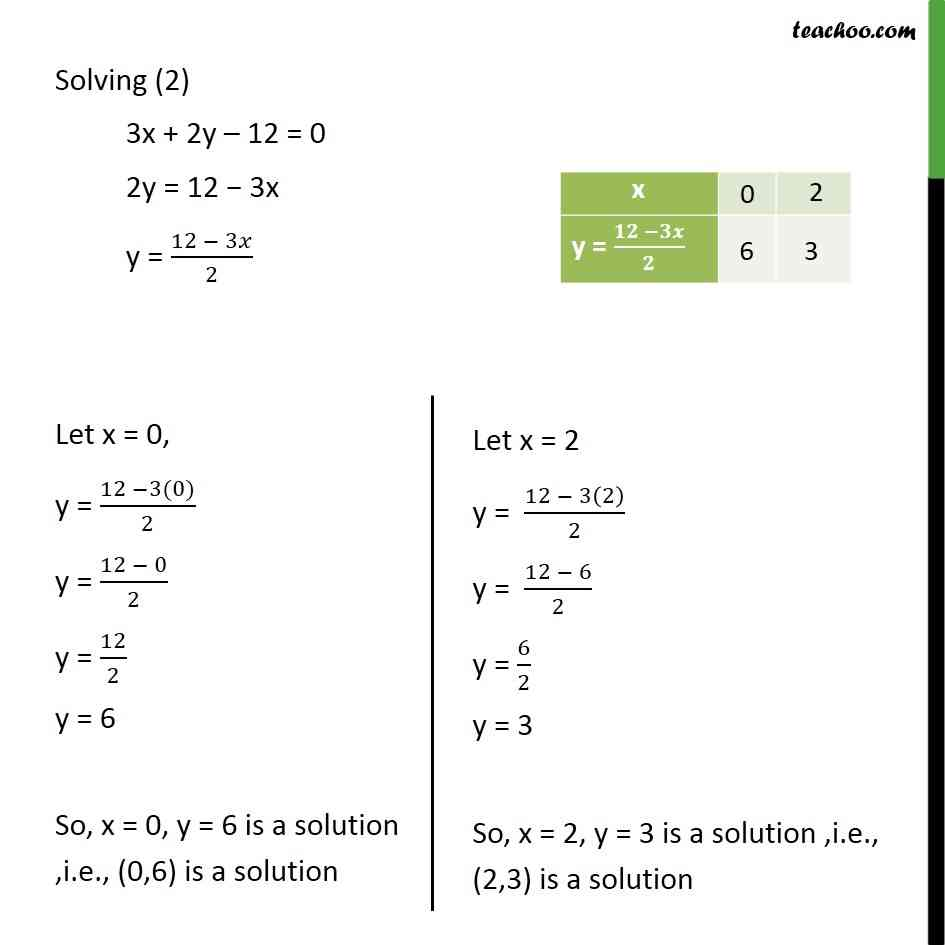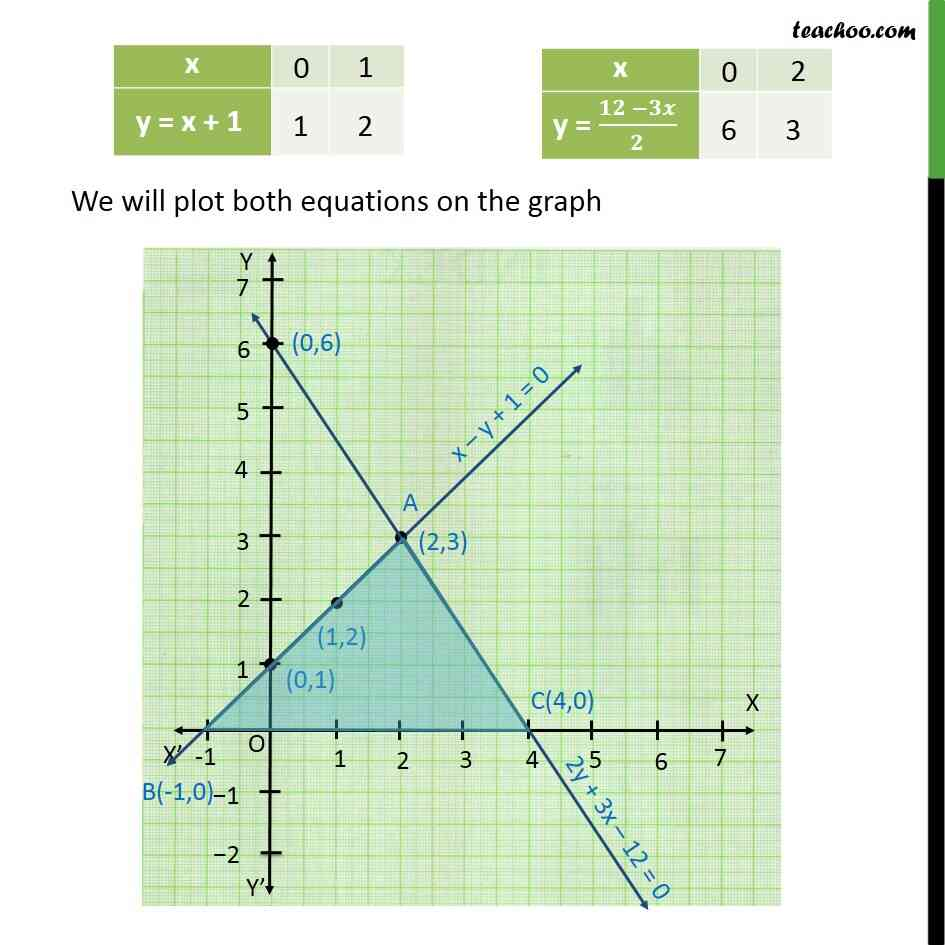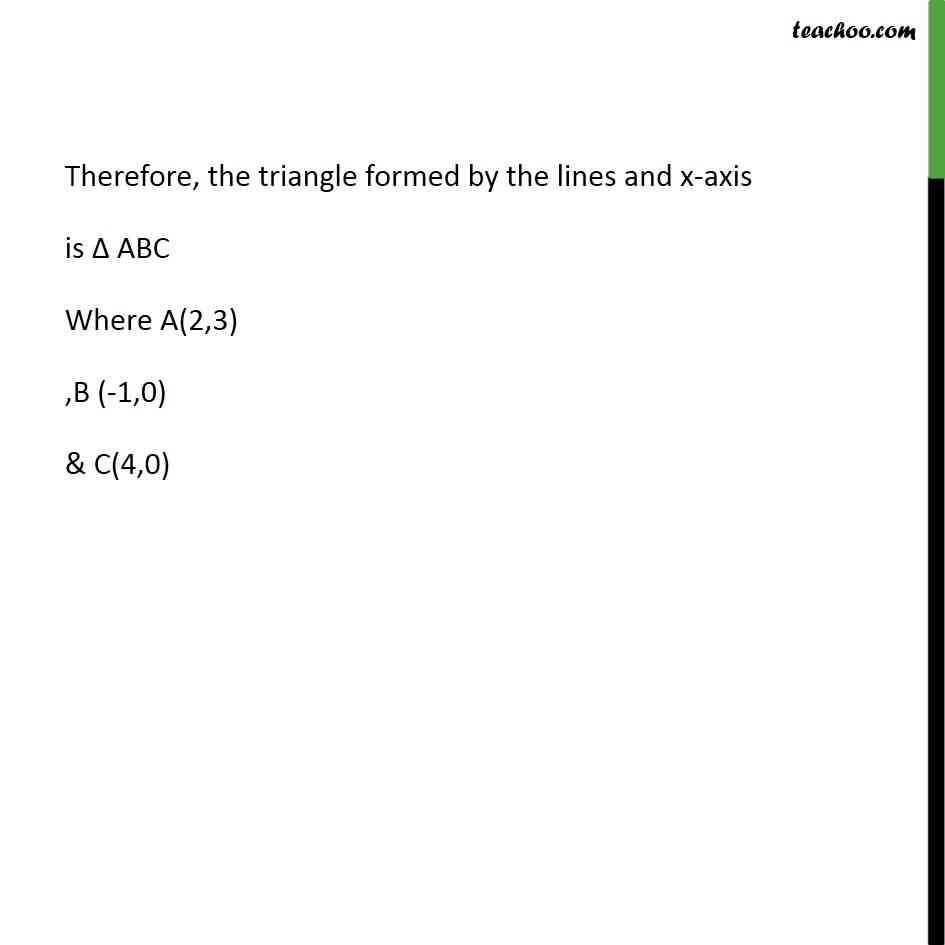1. Class 10
2. Important Questions for Exam - Class 10
3. Chapter 3 Class 10 Pair of Linear Equations in Two Variables

Transcript

Ex 3.2, 7 Draw the graphs of the equations x y + 1 = 0 and 3x+ 2y 12 = 0. Determine the coordinates of the vertices of the triangle formed by these lines and the x-axis, and shade the triangular region. x y + 1 = 0 3x + 2y 12 = 0 Solving (1) x y + 1 = 0 y = x + 1 Solving (2) 3x + 2y 12 = 0 2y = 12 3x y = (12 3 )/2

Chapter 3 Class 10 Pair of Linear Equations in Two Variables

Class 10
Important Questions for Exam - Class 10